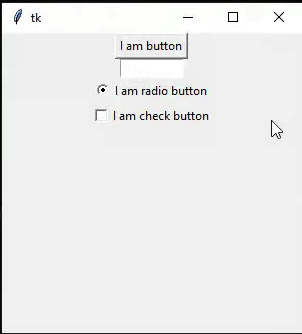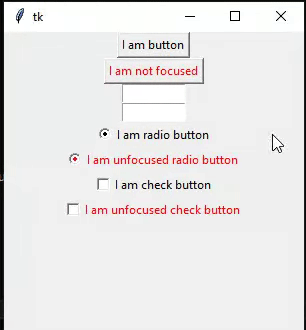GFG App
Open AppBrowser
Continue

# Disable focus for tkinter widgets – Python

Prerequisite: Python-Tkinter

In this article, we will discuss how to disable the focus from the widgets in the Tkinter framework. For disabling the focus, we use takefocus option inside the widget and assign its value to 0.

### Stepwise implementation:

Step1: Import Tkinter and initialize tkinter window

## Python3

 `# import tkinter  ` `import` `tkinter as tk ` ` `  `# initialize a tkinter window ` `root ``=` `tk.Tk() ` ` `  `# Creating the geometry with width and height of 300px ` `root.geometry(``"300x300"``) ` ` `  `# creating the body loop ` `root.mainloop()`

Step 2: Add some widgets to the application

# Creating button widget

btn = tk.Button(root, text=”Button”) # initializing the widget.

btn.pack() # calling the widget in application

Code:

## Python3

 `import` `tkinter as tk ` `  `  `# initialize a tkinter window ` `root ``=` `tk.Tk() ` ` `  `# Creating the geometry with width and height of 300px ` `root.geometry(``"300x300"``) ` ` `  `# create a button ` `btn ``=` `tk.Button(root, text ``=``"I am button"``) ` `btn.pack() ` ` `  `# text widget ` `txt ``=` `tk.Entry(root, width``=``10``) ` `txt.pack() ` ` `  `# create a radio button ` `rb ``=` `tk.Radiobutton(root, text ``=``"I am radio button"``)  ` `rb.pack()  ` ` `  `# create a check button ` `cb ``=` `tk.Checkbutton(root, text ``=` `"I am check button"``) ` `cb.pack() ` ` `  ` `  `root.mainloop()`

Output:

Here we notice that we are getting focus on each widget after pressing the TAB key.Step-3: Adding disable focus functionality to our program.

We use takefocus argument for disabling the focus

```Syntax: takefocus = 0

# for button
btn = tk.Button(root, text="Button", takefocus=0)```

The approach of the program is to disable the focus from the widgets with the help takefocus argument. We put its value to 0 for doing so. Other widgets have their focus enabled.

Code:

## Python3

 `import` `tkinter as tk ` `  `  `root ``=` `tk.Tk() ` `root.geometry(``"300x300"``) ` ` `  `# creating button ` `btn ``=` `tk.Button(root, text ``=``"I am button"``) ` `btn.pack() ` ` `  `# takefocus is set to 0 for disabling the TAB key ` `# focus in widget ` `btn_no_focus ``=` `tk.Button(root, text ``=``"I am not focused"``, ` `                         ``takefocus ``=` `0``,foreground ``=` `"red"``) ` `btn_no_focus.pack() ` ` `  `# created an entry widget with width 10 ` `txt ``=` `tk.Entry(root, width ``=` `10``) ` `txt.pack() ` ` `  `# creating a entry widget with width 10 and focus is ` `# 0 hence disabled  ` `txt ``=` `tk.Entry(root, width ``=` `10``, takefocus ``=` `0``) ` `txt.pack() ` ` `  `# Creating radiobutton ` `rb ``=` `tk.Radiobutton(root, text ``=``"I am radio button"``)  ` `rb.pack()  ` ` `  `# putting an takefocus=0 for disabling focus ` `rb_unfocus ``=` `tk.Radiobutton(root, text ``=``"I am unfocused radio button"``,  ` `                            ``takefocus ``=` `0``,foreground ``=` `"red"``)  ` `rb_unfocus.pack() ` ` `  `# creating the checkbutton ` `cb ``=` `tk.Checkbutton(root, text ``=` `"I am check button"``) ` `cb.pack() ` ` `  ` `  `cb_unfocused ``=` `tk.Checkbutton(root, text ``=` `"I am unfocused check button"``,  ` `                              ``takefocus ``=` `0``, foreground ``=` `"red"``) ` `cb_unfocused.pack() ` ` `  `root.mainloop()`

Output:

Red-colored widgets have takefocus=0 and others don’t have this argument. The red color is only used for clarity you may also remove it.My Personal Notes arrow_drop_up